# Probability Predictions

A probability prediction for an outcome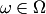is a probability measure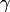on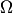. This is a task of probability forecasting. The quality of the probability predictionis often measured by the minus log-likelihood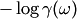(in the discrete case) or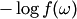(in the continuous case, where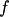is the probability density function of). Another popular loss function in the case of finiteis the Brier loss function. For other kinds of predictions, see game of prediction.